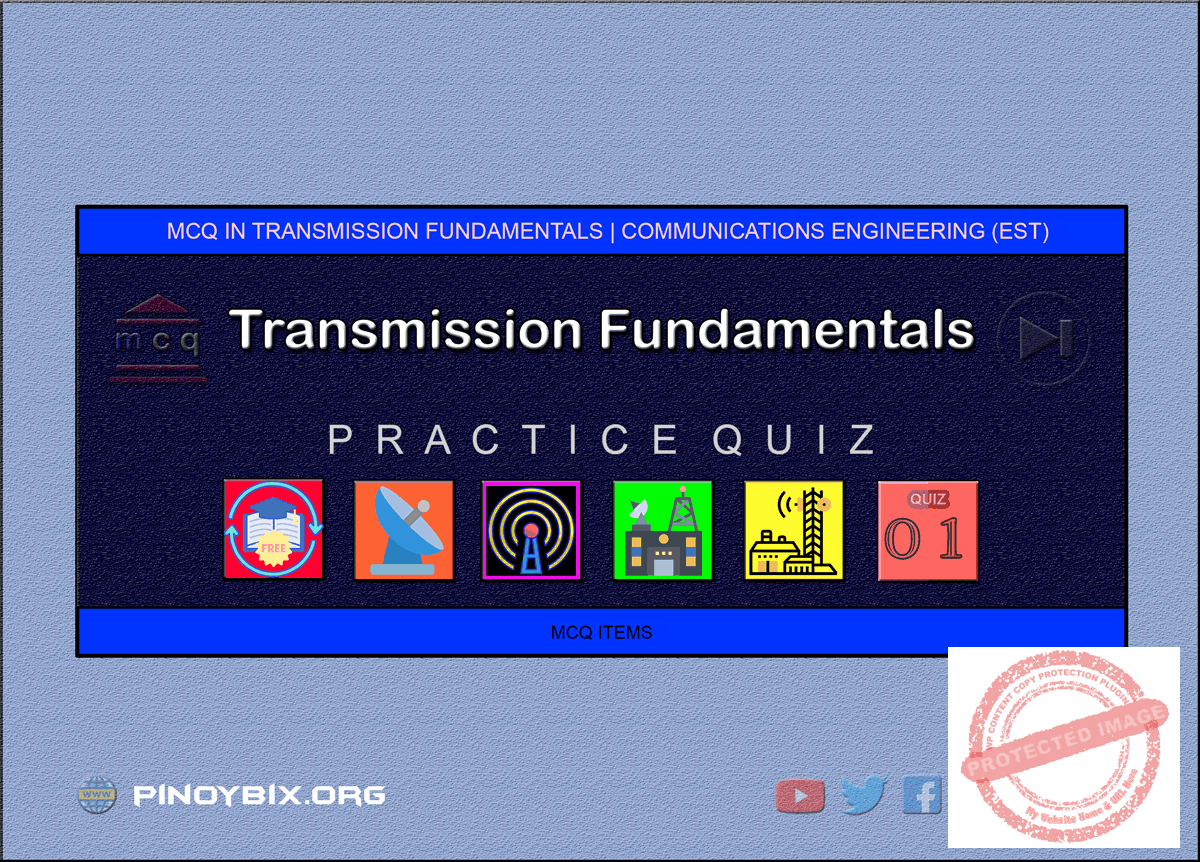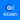# MCQ in Transmission Fundamentals Part 1 | ECE Board Exam

(Last Updated On: May 6, 2020)This is the Multiples Choice Questions Part 1 of the Series in Transmission Fundamentals as one of the Communications Engineering topic. In Preparation for the ECE Board Exam make sure to expose yourself and familiarize in each and every questions compiled here taken from various sources including but not limited to past Board Examination Questions in Electronic System and Technologies, Communications Books, Journals and other Communications References.

#### MCQ Topic Outline included in ECE Board Exam Syllabi

• MCQ in Transmission System
• MCQ in Transmission Medium
• MCQ in Velocity and Line Wavelength
• MCQ in Characteristics Impedance
• MCQ in Primary Line Constants and Propagation Constants
• MCQ in Standing Waves
• MCQ in Voltage Standing Wave Ratio
• MCQ in Telephone Lines and Cables
• MCQ in Wave Guides
• MCQ in Balanced and Unbalanced Lines
• MCQ in Twisted pair wire, Coaxial Cable
• MCQ in Decibel
• MCQ in Signal and Noise Fundamentals

#### Start Practice Exam Test Questions Part 1 of the Series

1. What is the opposition to the transfer of energy which is considered the dominant characteristic of a cable or circuit that emanates from its physical structure?

a. Conductance

b. Resistance

c. Reactance

d. Impedance

Solution:

2. When load impedance equals to Zo of the line, it means that the load _____ all the power.

a. reflects

b. absorbs

c. attenuates

Solution:

3. impedance matching ratio of a coax balun.

a. 1:4

b. 4:1

c. 2:1

d. 3:2

Solution:

4. Which stands for dB relative level?

a. dBrn

b. dBa

c. dBr

d. dBx

Solution:

5. Standard test tone used for audio measurement.

a. 800 Hz

b. 300 Hz

c. 100 Hz

d. 1000 Hz

Solution:

6. When VSWR is equal to zero, this means

a. that no power is applied

b. that the load is purely resistive

c. that the load is a pure reactance

d. that the load is opened

Solution:

7. _______ is the ratio of reflected voltage to the forward travelling voltage.

a. SWR

b. VSWR

c. Reflection coefficient

d. ISWR

Solution:

8. Transmission line must be matched to the load to ______.

a. transfer maximum voltage to the load

b. transfer maximum power to the load

d. transfer maximum current to the load

Solution:

9. Which indicate the relative energy loss in a capacitor?

a. Quality factor

b. Reactive factor

c. Dissipation factor

d. Power factor

Solution:

10. What is the standard test tone?

a. 0 dB

b. 0 dBW

c. 0 dBm

d. 0 dBrn

Solution:

11. The energy that neither radiated into space nor completely transmitted.

a. Reflected waves

b. Captured waves

c. Incident waves

d. Standing waves

Solution:

12. Micron is equal to _______ meter.

a. 10^-10

b. 10^-9

c. 10^-6

d. 10^-3

Solution:

13. 1 Angstrom (A) is equal to _______.

a. 10^-3 micron

b. 10^-10 m

c. 10^-6 micron

d. 10^-6 m

Solution:

14. Why is it impossible to use a waveguide at low radio frequencies?

a. Because of the size of the waveguide

b. Due to severe attenuation

c. Due to too much radiation

d. All of these

Solution:

15. ________ is the transmission and reception of information.

a. Modulation

b. Communications

d. Emission

Solution:

16. What is the loss of the circuit in dB if the power ration of output to input is 0.01?

a. 20

b. -20

c. 40

d. -40

Solution:

17. Transmission lines are either balanced or unbalanced with respect to

a. Negative terminal

b. Reference

c. Ground

d. Positive terminal

Solution:

18. The standing wave ratio is equal to _______ if the load is properly matched with the transmission line.

a. Infinity

b. 0

c. -1

d. 1

Solution:

19. ________ is the advantage of the balanced transmission line compared to unbalanced line.

a. Low attenuation

b. Easy installation

d. Tensile strength

Solution:

20. _______ is the method of determining the bandwidth of any processing system.

a. Fourier series

b. Spectral analysis

c. Frequency analysis

d. Bandwidth analysis

Solution:

21. What causes the attenuation present in a waveguide?

a. The air dielectric filling the guide

b. The coating of silver inside

c. Losses in the conducting walls of the guide

Solution:

22. A device that converts a balanced line to an unbalanced line of a transmission line.

a. Hybrid

b. Stub

c. Directional coupler

d. Balun

Solution:

23. What is the approximate line impedance of a parallel-strip line spaced 1 cm apart with the length of 50 cm?

a. 10 ohms

b. 15 ohms

c. 18 ohms

d. 23 ohms

Solution:

24. What is the average power rating of RG-58 C/u?

a. 25 W

b. 50 W

c. 75 W

d. 200 W

Solution:

25. A coaxial cable used for high temperatures.

a. RG-58C

b. RG-11A

c. RG-213

d. RG-211

Solution:

26. If you have available number of power amplifiers with a gain of 100 each, how many such amplifiers do you need to cascade to give an overall gain of 60dB?

a. 2

b. 3

c. 4

d. 5

Solution:

27. You are measuring noise in a voice channel at a -4 dB test point level, the meter reads -73 dBm, convert the reading into dBrnCO.

a. 12

b. 16

c. 18

d. 21

Solution:

28. The velocity factor for a transmission line

a. depends on the dielectric constant of the material used

b. increases the velocity along the transmission line

c. is governed by the skin effect

d. is higher for a solid dielectric than for air

Solution:

29. Impedance inversion can be obtained by

a. a short-circuited stub

b. an open-circuited stub

c. a quarter-wave line

d. a half-wave line

Solution:

30. Transmission lines when connected to antennas have

b. resistive load whose resistance is greater than the characteristic impedance of the line

c. resistive load whose resistance is less than the characteristic impedance of the line

d. resistive load at the resonant frequency

Solution:

31. One of the following is not a bounded media.

a. Coaxial line

b. Two-wire line

c. Waveguide

d. Ocean

Solution:

32. The impedance measured at the input of the transmission line when its length is infinite.

a. Input impedance

b. Open circuit impedance

c. Characteristic impedance

d. Short circuit impedance

Solution:

33. The following are considered primary line constants except

a. conductance

b. resistance

c. capacitance

d. complex propagation constant

Solution:

34. The dielectric constants of materials commonly used in transmission lines range from about

a. 1.2 to 2.8

b. 2.8 to 3.5

c. 3.5 to 5.2

d. 1.0 to 1.2

Solution:

35. Typically, the velocity factor (VF) of the materials used in transmission lines range from

a. 0.6 to 0.9

b. 0.1 to 0.5

c. 1.0 to 0.9

d. 0.6 to 0.8

Solution:

36. For an air dielectric two-wire line, the minimum characteristic impedance value is

a. 95 ohms

b. 85 ohms

c. 90 ohms

d. 88 ohms

Solution:

37. When a quarter-wave section transmission line is terminated by a short circuit and is connected to an RF source at the other end, its input impedance is

a. inductive

b. capacitive

c. resistive

d. equivalent to a parallel resonant LC circuit

Solution:

38. A transmitter operating at 30 MHz with 4 W output is connected via 10 m of RG-8A/u cable to an antenna that has an input resistance of 300 ohms. Find the reflection coefficient.

a. 0.71

b. 0.77

c. 0.97

d. 0.76

Solution:

39. A quarter wave transformer is connected to a parallel wire line in order to match the line to a load of 1000 ohms. The transformer has a characteristic impedance of 316.23 ohms. The distance between centers is 4 inches. What is the percentage reduction in the diameter of the line?

a. 85%

b. 83%

c. 86%

d. 90%

Solution:

40. The concept used to make one Smith chart universal is called

a. ionization

b. normalization

c. rationalization

d. termination

Solution:

41. What are the basic elements of communications system?

a. Source, transmission channel, transmitter

Solution:

42. ________ is the transmission of printed material over telephone lines.

a. Internet

b. Data communication

c. Telegraphy

d. Facsimile

Solution:

43. ________ is a continuous tone generated by the combination of two frequencies of 350 Hz and 440 Hz used in telephone sets.

a. DC tone

b. Ringing tone

c. Dial tone

d. Call waiting tone

Solution:

44. ________ are unidirectional amplifiers having 20-25 decibel gain that are placed about 75 km apart used to compensate for losses along the telephone line.

a. VF repeaters

c. Loop extenders

d. Echo suppressors

Solution:

45. ________ is a component in the telephone set that has the primary function of interfacing the handset to the local loop.

a. Resistor

b. Capacitor

c. Varistor

d. Induction coil

Solution:

46. Pulse dialing has ________ rate.

a. 20 pulses/min

b. 10 pulses/min

c. 10 pulses/sec

d. 80 pulses/sec

Solution:

47. ________ is a telephone wire that connects two central offices.

a. 2-wire circuit

b. Trunk line

c. Leased line

d. Private line

Solution:

48. The central switching office coordinating element for all cell sites that has cellular processor and a cellular switch. It interfaces with telephone company zone offices, control call processing and handle billing activities.

a. MTSO

b. Cell site

c. PTSN

d. Trunk line

Solution:

49. ________ in a cellular system performs radio-related functions for cellular site.

a. Switching system

b. Base station

c. Operation and support system

d. Mobile station

Solution:

50. A technology used to increase the capacity of a mobile phone system.

a. Frequency re-use

b. Cell splitting

c. TDM

d. FDM

Solution:

#### Questions and Answers in Transmission Fundamentals Series

Following is the list of multiple choice questions in this brand new series:

MCQ in Transmission Fundamentals
PART 1: MCQ from Number 1 – 50                        Answer key: PART 1
PART 2: MCQ from Number 51 – 100                   Answer key: PART 2
PART 3: MCQ from Number 101 – 150                 Answer key: PART 3
PART 4: MCQ from Number 151 – 200                 Answer key: PART 4
PART 5: MCQ from Number 201 – 250                 Answer key: PART 5
PART 6: MCQ from Number 251 – 300                 Answer key: PART 6
PART 7: MCQ from Number 301 – 350                 Answer key: PART 7
PART 8: MCQ from Number 351 – 400                 Answer key: PART 8
PART 9: MCQ from Number 401 – 450                 Answer key: PART 9
PART 10: MCQ from Number 451 – 500                Answer key: PART 10

### Complete List of MCQ in Communications Engineering per topic

Help Me Makes a Difference!

 P inoyBIX educates thousands of reviewers/students a day in preparation for their board examinations. Also provides professionals with materials for their lectures and practice exams. Help me go forward with the same spirit. “Will you make a small gift today via GCASH?”+63 966 459 6474 Option 1 : \$1 USD Option 2 : \$3 USD Option 3 : \$5 USD Option 4 : \$10 USD Option 5 : \$25 USD Option 6 : \$50 USD Option 7 : \$100 USD Option 8 : Other Amount© 2014 PinoyBIX Engineering. © 2019 All Rights Reserved | How to Donate? |